—— 莓鼻对罂粟霜，在她生产以后，《第四学徒》，第285页

Berrynose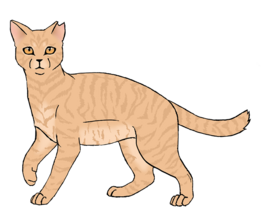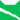雷族 (Q627)[临时][避难]雷族 (Q627)[注 1]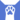反抗者 (Q4414)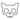鬼魂 (Q665)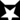无星之地 (Q635)[囚禁]雷族 武士雷族 副族长
（继任自松鼠飞 (Q3123)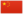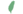莓、莓莓 Berry  莓、莓莓
 小莓 Berrykit  小莓
 莓爪 Berrypaw  莓掌
 莓鼻 Berrynose  莓鼻

Q2331：雷族公猫，原独行猫，自《黄昏战争》登场的角色

## 细节

### 外貌

• 他是一只身形结实的:89奶油色:猫物表长毛:11章公猫，:猫物表仅剩一小截又粗:14又短:18的尾巴。:169他的头颅宽大，:56肩膀宽阔，:198一只耳尖碎裂。:216

### 作者声明

• 维琪认为与蜜蕨相比，他最终还是更爱罂粟霜一些。毕竟他们真正组成了一个家庭并一同抚育了幼崽。

### 趣闻

• 他是继半尾之后第二只被断尾的猫。:猫物表
• 他成为副族长时并没有指导过学徒。:312

### 勘误

• 他曾被错误地描述为拥有和父亲及兄弟姐妹一样有灰白相间的皮毛。:240
• 在尾巴受伤后，他曾有几次被形容拥有完好的尾巴。:136:11、49:199、208

## 登场书目

• 黄昏战争 (Q143)[配角][首次登场🐱]
• 日落和平 (Q144)[配角]
• 预视力量 (Q152)[配角]
• 暗河汹涌 (Q153)[配角]
• 驱逐之战 (Q154)[配角]
• 天蚀遮月 (Q155)[配角]
• 暗夜长影 (Q156)[配角]
• 拂晓之光 (Q157)[配角]
• 第四学徒 (Q158)[配角]
• 战声渐近 (Q159)[配角]
• 暗夜密语 (Q160)[配角]
• 月光印记 (Q161)[配角]
• 武士归来 (Q162)[配角]
• 群星之战 (Q163)[配角]
• 学徒探索 (Q170)[配角]
• 雷影交加 (Q171)[配角]
• 天空破碎 (Q172)[配角]
• 极夜无光 (Q173)[配角]
• 烈焰焚河 (Q174)[配角]
• 风暴来袭 (Q175)[配角]
• 迷失群星 (Q3563)[配角]
• 静默冰融 (Q3579)[配角]
• 叠影重障 (Q3624)[配角][死亡时刻✝]
• 暗由心生 (Q4183)[对话提及]
• 无星之地 (Q4385)[配角]
• 迷雾明光 (Q4621)[配角]
• 河翻浪涌 (Q4643)[间接提及]
• 黑莓星的风暴 (Q182)[配角]
• 虎心的阴影 (Q185)[猫物闲角]
• 鸦羽的拷问 (Q186)[配角]
• 松鼠飞的希冀 (Q3561)[配角]
• 一星的告解 (Q4826)[配角]
• 冬青叶的故事 (Q187)[猫物闲角]
• 雾星的征兆 (Q188)[猫物闲角]
• 叶池的祈愿 (Q191)[配角]
• 鸽翅的沉默 (Q192)[配角]
• 褐皮的族群 (Q3566)[猫物闲角]
• 黛西的至亲 (Q3629)[对话提及]
• 斑毛的反抗 (Q4337)[配角]

1. 因放逐而离开

## 参考文献

这篇文章基于CC BY-SA 3.0许可使用了猫武士维基（英语）Berrynose一文中的部分内容。
1. 《叠影重障》第二十一章 (Q4521)
2. 《黄昏战争》第九章 (Q1031)
3. 《族群的秘密》（简体中文）.武汉出版社
4. 《部族解密》（繁体中文）.晨星出版社
5. 第四学徒 (Q158)
6. 日落和平
7. 预视力量
8. 迷失群星
9. 暗河汹涌
10. 驱逐之战
11. 群星之战
12. 天蚀遮月
13. Vicky Holmes (2015-07-16). 站内截图. Facebook . 原页面 归档于 2017-04-18.
14. 呼唤野性
15. 静默冰融
16. 武士归来
17. 叠影重障
1. 在同一份由维琪授意、Su所公布的黑森林名单中，官网家谱已将雪暴翅、亮花和沼云标注为星族成员，短尾标注为黑森林成员；鹿脚、百合须和缠果的死后去处则暂时没有更进一步的资讯。